# Search

About 85 Search Results Matching Types of Worksheet, Worksheet Section, Generator, Generator Section, Subjects matching Math, Type matching Worksheet, Grades matching 4th Grade, Similar to Easy Measurement Worksheets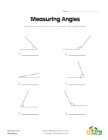## Measuring Angles Worksheet 1

Measure each of the angles with a protractor an...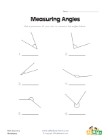## Measuring Angles Worksheet 2

Use a protractor to measure each of the angles.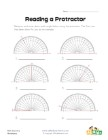## Reading a Protractor Worksheet 2

Read each protractor and write down the angles ...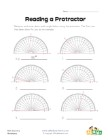## Reading a Protractor Worksheet 1

Read each protractor and write down the angles ...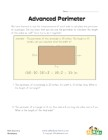Learn to use the perimeter to calculate the len...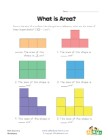## Beginner Area Worksheet

Use this worksheet as an introduction to calcul...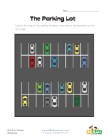## Parking Lot Story Problems Worksheet

Use the map of the cars in the parking lot to a...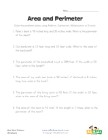## Area and Perimeter Word Problems Worksheet

Solve the 6 area and perimeter word problems in...